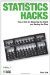# M

## Index

[SYMBOL] [A] [B] [C] [D] [E] [F] [G] [H] [I] [J] [K] [L] [M] [N] [O] [P] [Q] [R] [S] [T] [V] [W] [X] [Y] [Z]

magic number, lotteries and
MANOVA (multivariate analysis of variance)
ACT
calculating
Central Limit Theorem
central tendency and
cut score and 2nd
defined 2nd
effect size and
linear regression and
normal curve and 2nd
normal distribution
precision of
predicting test performance 2nd
regression toward 2nd 3rd
T scores
z score 2nd 3rd
<Emphasis>t</> tests
categorical
converting raw scores
defined
effect of increasing sample size
Gott's Principle
graphs and
improving test scores
levels of 2nd
normal distribution
percentile ranks
precise
predicting with normal curve
probability characteristics
reliability of
standardized scores 2nd
testing fairly
validity of 2nd 3rd
measures of central tendency
median
central tendency and 2nd 3rd
defined
normal curve and
medical decisions
Michie, Donald
Microsoft Excel
DATAS software
histograms
predicting football games
Milgram, Stanley 2nd 3rd 4th
mind control
Minnesota Multiphase Personality Inventory-II test
mnemonic devices
mode
central tendency and 2nd
defined
normal curve and
models
building 2nd
defined
goodness-of-fit statistic and
money
casinos and 2nd
infinite doubling of
Monopoly
Monty Hall problem
multiple choice questions
writing good 2nd 3rd
multiple regression
criterion variables and
defined
multiple predictor variables
predicting football games
multiple regression)
multiplicative rule 2nd
multivariate analysis of variance (MANOVA)
mutually exclusive outcomesStatistics Hacks: Tips & Tools for Measuring the World and Beating the Odds
ISBN: 0596101643
EAN: 2147483647
Year: 2004
Pages: 114
Authors: Bruce Frey

Similar book on Amazon